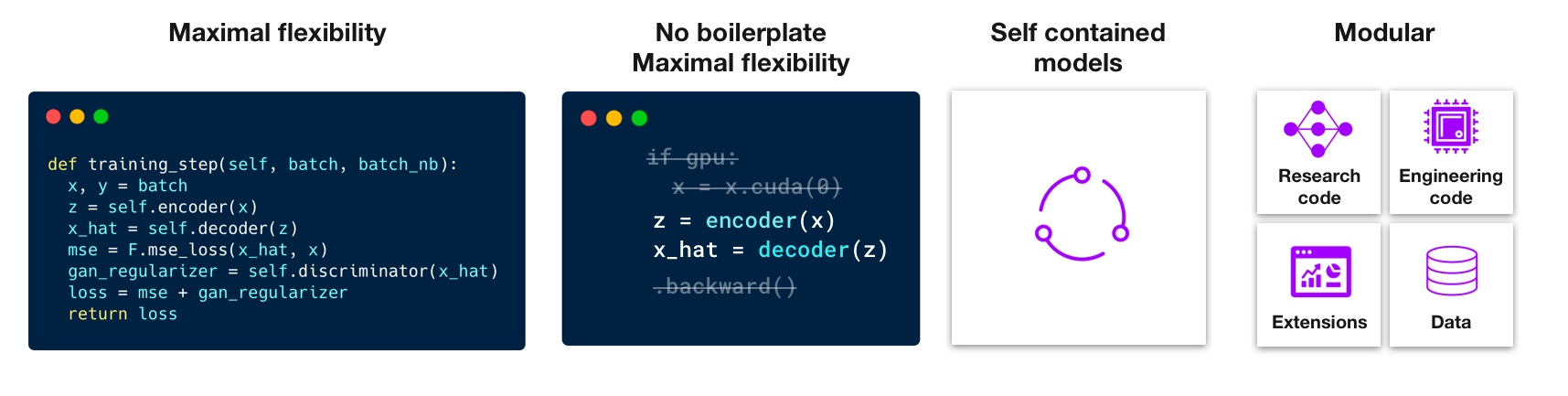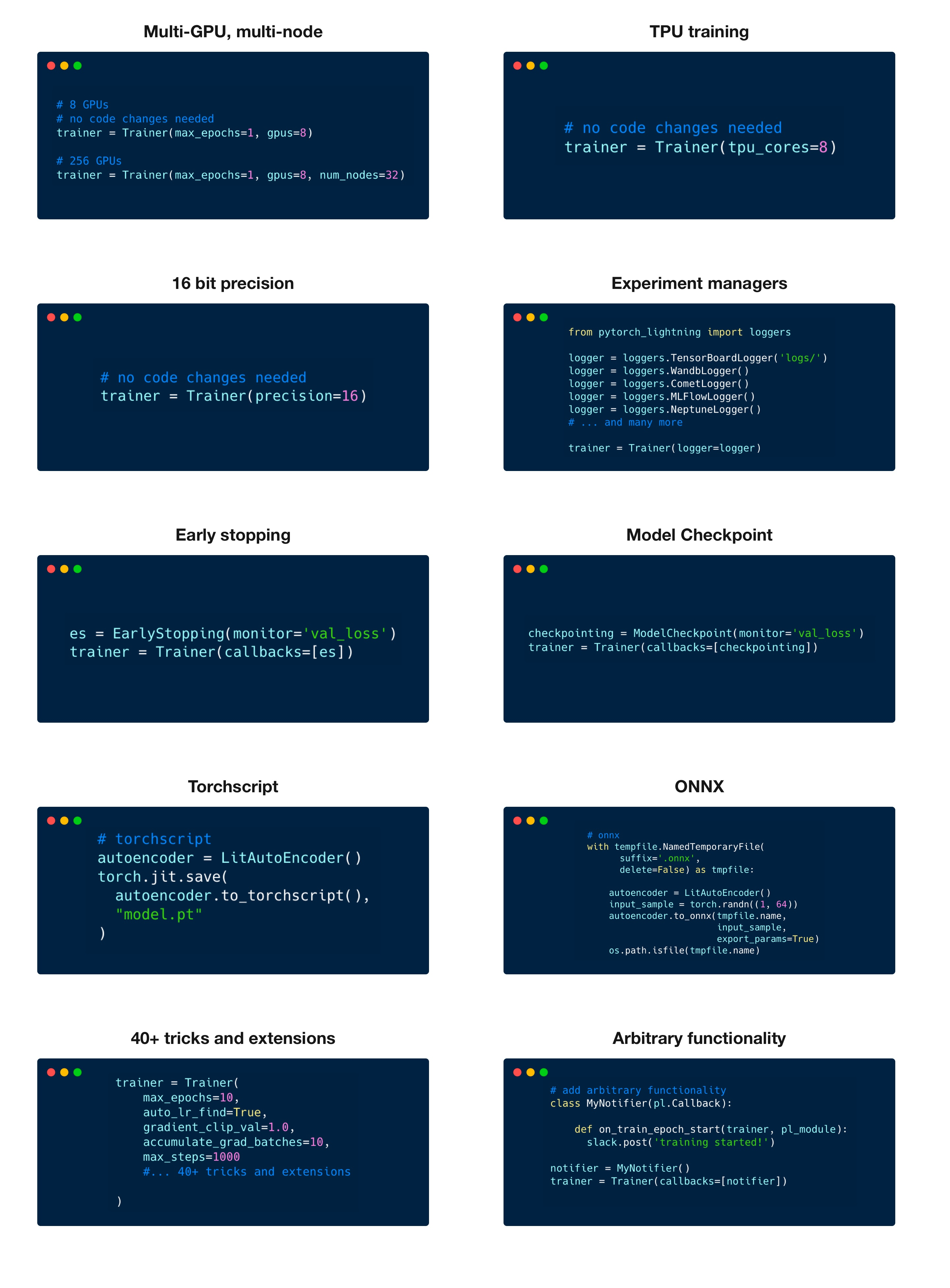# loongson/pypi/: pytorch-lightning-1.5.1 metadata and description

PyTorch Lightning is the lightweight PyTorch wrapper for ML researchers. Scale your models. Write less boilerplate.

 author William Falcon et al. author_email waf2107@columbia.edu classifiers Environment :: Console Natural Language :: English Development Status :: 4 - Beta Intended Audience :: Developers Topic :: Scientific/Engineering :: Artificial Intelligence Topic :: Scientific/Engineering :: Image Recognition Topic :: Scientific/Engineering :: Information Analysis License :: OSI Approved :: Apache Software License Operating System :: OS Independent Programming Language :: Python :: 3 Programming Language :: Python :: 3.6 Programming Language :: Python :: 3.7 Programming Language :: Python :: 3.8 Programming Language :: Python :: 3.9 description_content_type text/markdown download_url https://github.com/PyTorchLightning/pytorch-lightning keywords deep learning,pytorch,AI license Apache-2.0 platform UNKNOWN project_urls Bug Tracker, https://github.com/PyTorchLightning/pytorch-lightning/issues Documentation, https://pytorch-lightning.rtfd.io/en/latest/ Source Code, https://github.com/PyTorchLightning/pytorch-lightning provides_extras test requires_dist numpy (>=1.17.2) torch (>=1.6) future (>=0.17.1) tqdm (>=4.41.0) PyYAML (>=5.1) fsspec[http] (!=2021.06.0,>=2021.05.0) tensorboard (>=2.2.0) torchmetrics (>=0.4.1) pyDeprecate (==0.3.1) packaging (>=17.0) typing-extensions matplotlib (>3.1) ; extra == 'all' horovod (>=0.21.2) ; extra == 'all' torchtext (>=0.7) ; extra == 'all' omegaconf (>=2.0.5) ; extra == 'all' hydra-core (>=1.0.5) ; extra == 'all' jsonargparse[signatures] (>=3.19.3) ; extra == 'all' gcsfs (>=2021.5.0) ; extra == 'all' rich (>=10.2.2) ; extra == 'all' neptune-client (>=0.10.0) ; extra == 'all' comet-ml (>=3.1.12) ; extra == 'all' mlflow (>=1.0.0) ; extra == 'all' test-tube (>=0.7.5) ; extra == 'all' wandb (>=0.8.21) ; extra == 'all' coverage (>5.2.0) ; extra == 'all' codecov (>=2.1) ; extra == 'all' pytest (>=6.0) ; extra == 'all' pytest-rerunfailures (>=10.2) ; extra == 'all' check-manifest ; extra == 'all' twine (==3.2) ; extra == 'all' mypy (>=0.900) ; extra == 'all' flake8 (>=3.9.2) ; extra == 'all' pre-commit (>=1.0) ; extra == 'all' cloudpickle (>=1.3) ; extra == 'all' scikit-learn (>0.22.1) ; extra == 'all' onnxruntime ; extra == 'all' pandas ; extra == 'all' torchvision (>=0.7) ; extra == 'all' gym (>=0.17.0) ; extra == 'all' ipython[all] ; extra == 'all' matplotlib (>3.1) ; extra == 'cpu' torchtext (>=0.7) ; extra == 'cpu' omegaconf (>=2.0.5) ; extra == 'cpu' hydra-core (>=1.0.5) ; extra == 'cpu' jsonargparse[signatures] (>=3.19.3) ; extra == 'cpu' gcsfs (>=2021.5.0) ; extra == 'cpu' rich (>=10.2.2) ; extra == 'cpu' neptune-client (>=0.10.0) ; extra == 'cpu' comet-ml (>=3.1.12) ; extra == 'cpu' mlflow (>=1.0.0) ; extra == 'cpu' test-tube (>=0.7.5) ; extra == 'cpu' wandb (>=0.8.21) ; extra == 'cpu' coverage (>5.2.0) ; extra == 'cpu' codecov (>=2.1) ; extra == 'cpu' pytest (>=6.0) ; extra == 'cpu' pytest-rerunfailures (>=10.2) ; extra == 'cpu' check-manifest ; extra == 'cpu' twine (==3.2) ; extra == 'cpu' mypy (>=0.900) ; extra == 'cpu' flake8 (>=3.9.2) ; extra == 'cpu' pre-commit (>=1.0) ; extra == 'cpu' cloudpickle (>=1.3) ; extra == 'cpu' scikit-learn (>0.22.1) ; extra == 'cpu' onnxruntime ; extra == 'cpu' pandas ; extra == 'cpu' torchvision (>=0.7) ; extra == 'cpu' gym (>=0.17.0) ; extra == 'cpu' ipython[all] ; extra == 'cpu' matplotlib (>3.1) ; extra == 'cpu-extra' torchtext (>=0.7) ; extra == 'cpu-extra' omegaconf (>=2.0.5) ; extra == 'cpu-extra' hydra-core (>=1.0.5) ; extra == 'cpu-extra' jsonargparse[signatures] (>=3.19.3) ; extra == 'cpu-extra' gcsfs (>=2021.5.0) ; extra == 'cpu-extra' rich (>=10.2.2) ; extra == 'cpu-extra' matplotlib (>3.1) ; extra == 'dev' horovod (>=0.21.2) ; extra == 'dev' torchtext (>=0.7) ; extra == 'dev' omegaconf (>=2.0.5) ; extra == 'dev' hydra-core (>=1.0.5) ; extra == 'dev' jsonargparse[signatures] (>=3.19.3) ; extra == 'dev' gcsfs (>=2021.5.0) ; extra == 'dev' rich (>=10.2.2) ; extra == 'dev' neptune-client (>=0.10.0) ; extra == 'dev' comet-ml (>=3.1.12) ; extra == 'dev' mlflow (>=1.0.0) ; extra == 'dev' test-tube (>=0.7.5) ; extra == 'dev' wandb (>=0.8.21) ; extra == 'dev' coverage (>5.2.0) ; extra == 'dev' codecov (>=2.1) ; extra == 'dev' pytest (>=6.0) ; extra == 'dev' pytest-rerunfailures (>=10.2) ; extra == 'dev' check-manifest ; extra == 'dev' twine (==3.2) ; extra == 'dev' mypy (>=0.900) ; extra == 'dev' flake8 (>=3.9.2) ; extra == 'dev' pre-commit (>=1.0) ; extra == 'dev' cloudpickle (>=1.3) ; extra == 'dev' scikit-learn (>0.22.1) ; extra == 'dev' onnxruntime ; extra == 'dev' pandas ; extra == 'dev' torchvision (>=0.7) ; extra == 'examples' gym (>=0.17.0) ; extra == 'examples' ipython[all] ; extra == 'examples' matplotlib (>3.1) ; extra == 'extra' horovod (>=0.21.2) ; extra == 'extra' torchtext (>=0.7) ; extra == 'extra' omegaconf (>=2.0.5) ; extra == 'extra' hydra-core (>=1.0.5) ; extra == 'extra' jsonargparse[signatures] (>=3.19.3) ; extra == 'extra' gcsfs (>=2021.5.0) ; extra == 'extra' rich (>=10.2.2) ; extra == 'extra' neptune-client (>=0.10.0) ; extra == 'loggers' comet-ml (>=3.1.12) ; extra == 'loggers' mlflow (>=1.0.0) ; extra == 'loggers' test-tube (>=0.7.5) ; extra == 'loggers' wandb (>=0.8.21) ; extra == 'loggers' coverage (>5.2.0) ; extra == 'test' codecov (>=2.1) ; extra == 'test' pytest (>=6.0) ; extra == 'test' pytest-rerunfailures (>=10.2) ; extra == 'test' check-manifest ; extra == 'test' twine (==3.2) ; extra == 'test' mypy (>=0.900) ; extra == 'test' flake8 (>=3.9.2) ; extra == 'test' pre-commit (>=1.0) ; extra == 'test' cloudpickle (>=1.3) ; extra == 'test' scikit-learn (>0.22.1) ; extra == 'test' onnxruntime ; extra == 'test' pandas ; extra == 'test' requires_python >=3.6

Because this project isn't in the `mirror_whitelist`, no releases from root/pypi are included.

File Tox results History
pytorch_lightning-1.5.1-py3-none-any.whl
Size
1011 KB
Type
Python Wheel
Python
3
• Replaced 1 time(s)
• Uploaded to loongson/pypi by loongson 2022-09-06 07:44:51The lightweight PyTorch wrapper for high-performance AI research. Scale your models, not the boilerplate.

## PyTorch Lightning is just organized PyTorch

Lightning disentangles PyTorch code to decouple the science from the engineering.

## Lightning Design Philosophy

Lightning structures PyTorch code with these principles:Lightning forces the following structure to your code which makes it reusable and shareable:

• Research code (the LightningModule).
• Engineering code (you delete, and is handled by the Trainer).
• Non-essential research code (logging, etc... this goes in Callbacks).
• Data (use PyTorch DataLoaders or organize them into a LightningDataModule).

Once you do this, you can train on multiple-GPUs, TPUs, CPUs and even in 16-bit precision without changing your code!

Get started with our 2 step guide

## Continuous Integration

Lightning is rigorously tested across multiple GPUs, TPUs CPUs and against major Python and PyTorch versions.

Current build statuses <center>
• ** tests run on two NVIDIA P100
• *** tests run on Google GKE TPUv2/3
• TPU py3.7 means we support Colab and Kaggle env.
</center>

## How To Use

### Step 0: Install

Simple installation from PyPI

```pip install pytorch-lightning
```

### Step 1: Add these imports

```import os
import torch
from torch import nn
import torch.nn.functional as F
from torchvision.datasets import MNIST
from torchvision import transforms
import pytorch_lightning as pl
```

### Step 2: Define a LightningModule (nn.Module subclass)

A LightningModule defines a full system (ie: a GAN, autoencoder, BERT or a simple Image Classifier).

```class LitAutoEncoder(pl.LightningModule):
def __init__(self):
super().__init__()
self.encoder = nn.Sequential(nn.Linear(28 * 28, 128), nn.ReLU(), nn.Linear(128, 3))
self.decoder = nn.Sequential(nn.Linear(3, 128), nn.ReLU(), nn.Linear(128, 28 * 28))

def forward(self, x):
# in lightning, forward defines the prediction/inference actions
embedding = self.encoder(x)
return embedding

def training_step(self, batch, batch_idx):
# training_step defines the train loop. It is independent of forward
x, y = batch
x = x.view(x.size(0), -1)
z = self.encoder(x)
x_hat = self.decoder(z)
loss = F.mse_loss(x_hat, x)
self.log("train_loss", loss)
return loss

def configure_optimizers(self):
return optimizer
```

Note: Training_step defines the training loop. Forward defines how the LightningModule behaves during inference/prediction.

### Step 3: Train!

```dataset = MNIST(os.getcwd(), download=True, transform=transforms.ToTensor())
train, val = random_split(dataset, [55000, 5000])

autoencoder = LitAutoEncoder()
trainer = pl.Trainer()
```

Lightning has over 40+ advanced features designed for professional AI research at scale.

Here are some examples:Highlighted feature code snippets
```# 8 GPUs
# no code changes needed
trainer = Trainer(max_epochs=1, gpus=8)

# 256 GPUs
trainer = Trainer(max_epochs=1, gpus=8, num_nodes=32)
```
Train on TPUs without code changes
```# no code changes needed
trainer = Trainer(tpu_cores=8)
```
16-bit precision
```# no code changes needed
trainer = Trainer(precision=16)
```
Experiment managers
```from pytorch_lightning import loggers

# tensorboard
trainer = Trainer(logger=TensorBoardLogger("logs/"))

# weights and biases
trainer = Trainer(logger=loggers.WandbLogger())

# comet
trainer = Trainer(logger=loggers.CometLogger())

# mlflow
trainer = Trainer(logger=loggers.MLFlowLogger())

# neptune
trainer = Trainer(logger=loggers.NeptuneLogger())

# ... and dozens more
```
EarlyStopping
```es = EarlyStopping(monitor="val_loss")
trainer = Trainer(callbacks=[es])
```
Checkpointing
```checkpointing = ModelCheckpoint(monitor="val_loss")
trainer = Trainer(callbacks=[checkpointing])
```
Export to torchscript (JIT) (production use)
```# torchscript
autoencoder = LitAutoEncoder()
torch.jit.save(autoencoder.to_torchscript(), "model.pt")
```
Export to ONNX (production use)
```# onnx
with tempfile.NamedTemporaryFile(suffix=".onnx", delete=False) as tmpfile:
autoencoder = LitAutoEncoder()
input_sample = torch.randn((1, 64))
autoencoder.to_onnx(tmpfile.name, input_sample, export_params=True)
os.path.isfile(tmpfile.name)
```

### Pro-level control of training loops (advanced users)

For complex/professional level work, you have optional full control of the training loop and optimizers.

```class LitAutoEncoder(pl.LightningModule):
def __init__(self):
super().__init__()
self.automatic_optimization = False

def training_step(self, batch, batch_idx):
# access your optimizers with use_pl_optimizer=False. Default is True
opt_a, opt_b = self.optimizers(use_pl_optimizer=True)

loss_a = ...
self.manual_backward(loss_a, opt_a)
opt_a.step()

loss_b = ...
self.manual_backward(loss_b, opt_b, retain_graph=True)
self.manual_backward(loss_b, opt_b)
opt_b.step()
```

• Models become hardware agnostic
• Code is clear to read because engineering code is abstracted away
• Easier to reproduce
• Make fewer mistakes because lightning handles the tricky engineering
• Keeps all the flexibility (LightningModules are still PyTorch modules), but removes a ton of boilerplate
• Lightning has dozens of integrations with popular machine learning tools.
• Tested rigorously with every new PR. We test every combination of PyTorch and Python supported versions, every OS, multi GPUs and even TPUs.
• Minimal running speed overhead (about 300 ms per epoch compared with pure PyTorch).

## Community

The lightning community is maintained by

• 10+ core contributors who are all a mix of professional engineers, Research Scientists, and Ph.D. students from top AI labs.
• 480+ active community contributors.

Want to help us build Lightning and reduce boilerplate for thousands of researchers? Learn how to make your first contribution here

Lightning is also part of the PyTorch ecosystem which requires projects to have solid testing, documentation and support.

If you have any questions please:

### Funding

We're venture funded to make sure we can provide around the clock support, hire a full-time staff, attend conferences, and move faster through implementing features you request.

## Grid AI

Grid AI is our platform for training models at scale on the cloud!

``````python my_model.py --learning_rate 1e-6 --layers 2 --gpus 4
``````grid train --grid_gpus 4 my_model.py --learning_rate 'uniform(1e-6, 1e-1, 20)' --layers '[2, 4, 8, 16]'